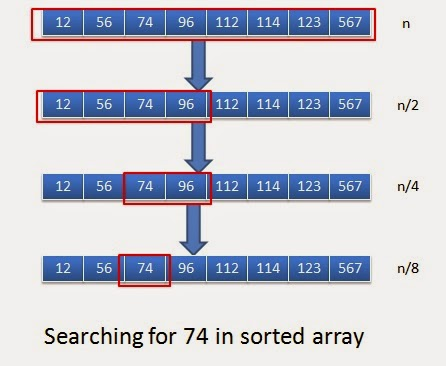# What is Logarithmic complexity?

### Logarithmic complexity

Lets understand logarithmic complexity with the help of example.You might know about binary search.When you want to find a value in sorted array, we use binary search.

1

2

3

4

5

6

7

8

9

10

11

12

13

14

15

16

17 public int binarySearch(int[] sorted, int first, int last, int elementToBeSearched) {

int iteration=0;

while (first < last) {

iteration++;

System.out.println(“i”+iteration);

int mid = (first + last) / 2; // Compute mid point.

System.out.println(mid);

if (elementToBeSearched < sorted[mid]) { last = mid; // repeat search in first half. } else if (elementToBeSearched > sorted[mid]) {

first = mid + 1; // Repeat search in last half.

} else {

return mid; // Found it. return position

}

}

return -1; // Failed to find element

}

Now let’s assume our soreted array is:

1

2

3 int[] sortedArray={12,56,74,96,112,114,123,567};

and we want to search for 74 in above array. Below diagram will explain how binary search will work here.When you observe closely, in each of the iteration you are cutting scope of array to the half. In every iteration, we are overriding value of first or last depending on soretedArray[mid].
So for
0th iteration : n
1th iteration: n/2
2nd iteration n/4
3rd iteration n/8.
Generalizing above equation:
For ith iteration : n/2i

So iteration will end , when we have 1 element left i.e. for any i, which will be our last iteration:
1=n/2i;
2i=n;
after taking log
i= log(n);
so it concludes that number of iteration requires to do binary search is log(n) so complexity of binary search is log(n)
It makes sense as in our example, we have n as 8 . It took 3 iterations(8->4->2->1) and 3 is log(8).
So If we are dividing input size by k in each iteration,then its complexity will be O(logk(n)) that is log(n) base k.

Lets take an example:

1

2

3

4

5

6

7 int m=0;

// executed log(n) times

for (int i = 0; i < n; i=i*2) {

m=m+1;

}

Exercise:

Lets do some exercise and find complexity of given code:

1
2
3
4
5
6

``````     int m=0;
for (int i = 0; i < n; i++) {
m=m+1;
``````

}

Ans:
1
2
3
4
5
6
7

``````     int m=0;
// Executed n times
for (int i = 0; i < n; i++) {
m=m+1;
``````

}

Complexity will be O(n)

1
2
3
4
5
6
7
8
9
10
11

``````    int m=0;
for (int i = 0; i < n; i++) {
m=m+1;
``````

}
for (int i = 0; i < n; i++) {
for(int j = 0; j < n; j++)
m=m+1;
}
}

Ans:
1
2
3
4
5
6
7
8
9
10
11
12
13

``````    int m=0;
// Executed n times
for (int i = 0; i < n; i++) {
m=m+1;
``````

}
// outer loop executed n times
for (int i = 0; i < n; i++) {
// inner loop executed n times
for(int j = 0; j < n; j++)
m=m+1;
}

1
2
3

}

Complexity will be :n+n*n —>O(n^2)

1
2
3
4
5
6
7
8
9
10
11
12
13

``````    int m=0;

// outer loop executed n times
``````

for (int i = 0; i < n; i++) {
// middle loop executed n/2 times
for(int j = n/2; j < n; j++)
for(int k=0;k*k < n; k++ )
m=m+1;
}
}
}

Ans:
1
2
3
4
5
6
7
8
9
10
11
12
13
14

``````    int m=0;

// outer loop executed n times
``````

for (int i = 0; i < n; i++) {
// middle loop executed n/2 times
for(int j = n/2; j < n; j++)
// inner loop executed log(n) times
for(int k=0;k*k < n; k++ )
m=m+1;
}
}
}

Complexity will be nn/2log(n)–> n^2log(n)

1
2
3
4
5
6
7
8
9
10

int m=0;

for (int i = n/2; i < n; i++) {
for(int j = n/2; j < n; j++)
for(int k=0;k < n; k++ )
m=m+1;
}

Ans:
1
2
3
4
5
6
7
8
9
10
11
12

int m=0;

``````// outer loop executed n/2 times
``````

for (int i = n/2; i < n; i++) {
// middle loop executed n/2 times
for(int j = n/2; j < n; j++)
// inner loop executed n times
for(int k=0;k < n; k++ )
m=m+1;
}

Big O log n time complexity (O(log n)) essentially means that the running time grows in proportion to the logarithm of the input size.

As an example,

If 10 items take at most some amount of time x,

And 100 items take at most, say, 2x,

And 10,000 items take at most 4x,

Then it’s looking like an O (log n) time. If a solution is taking Big O (log n) time complexity than it means it is slow. One can improve it’s time complexity by applying some other solution.

Binary search Algorithm is one of the algorithms with worst case time complexity as O (log n).